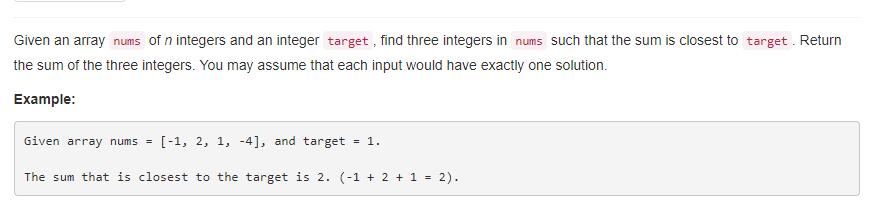# 题目描述（中等难度）# 解法一 暴力解法

public int threeSumClosest(int[] nums, int target) {
int sub = Integer.MAX_VALUE; //保存和 target 的差值
int sum = 0; //保存当前最接近 target 的三个数的和
for (int i = 0; i < nums.length; i++) {
for (int j = i + 1; j < nums.length; j++)
for (int k = j + 1; k < nums.length; k++) {
if (Math.abs((nums[i] + nums[j] + nums[k] - target)) < sub) {
sum = nums[i] + nums[j] + nums[k];
sub = Math.abs(sum - target);
}
}
}
return sum;
}


# 解法二

public int threeSumClosest(int[] nums, int target) {
Arrays.sort(nums);
int sub=Integer.MAX_VALUE;
int sum=0;
for(int i=0;i<nums.length;i++){
int lo=i+1,hi=nums.length-1;
while(lo<hi){
if(Math.abs((nums[lo]+nums[hi]+nums[i]-target))<sub){
sum=nums[lo]+nums[hi]+nums[i];
sub=Math.abs(sum-target);
}
if(nums[lo]+nums[hi]+nums[i]>target){
hi--;
}else{
lo++;
}
}
}
return sum;
}